Science
Technology |

# 10 Enormous Numbers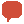. . . Comments

One of the first questions that kids often ask is “What is the biggest number?” This question is an important step in transitioning to a world of abstract concepts. The answer is of course that numbers are generally considered endless, but there gets to be a point were numbers become so big that there really is no point in having them, they have no real importance outside of the fact that yes technically they do exist. To make a list like this I could simply write down a massive number for the first number, and then write +1, +2, +3 and so on for the rest of the list. Instead I chose to take out 10 numbers that do have some effect on the world and place them in ascending order, giving a brief explanation as to what they are and how they have some relevance on the world, albeit very small relevance, especially when compared to the size of the number itself.

10

10^80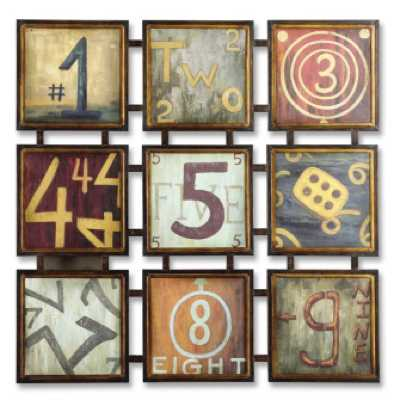Ten to the eightieth power – a 1 with 80 zeros after it – is quite massive but somewhat tangible at least from a relatively concrete point of view. This is the estimated number of fundamental particles in the known universe, and with fundamental particles we’re not talking about microscopic particles, we’re talking about much smaller things like Quarks and Leptons – subatomic particles. The name for this number in U.S. and Modern British is “One Hundred Quinquavigintillion” I would write out phonetically how to pronounce that but I don’t have a clue. The concept of the amount of such small things and how many of them make up the entire universe may seem overwhelming, but it is the smallest and easiest to understand of the numbers on this list.

9

One Googol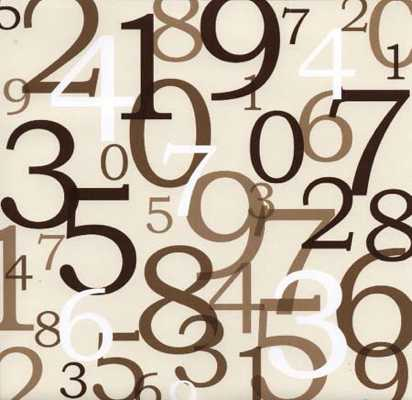The word googol, with a slightly different spelling, has become a frequently used verb in modern times, thanks to a highly popular search engine. The number has an interesting history which you can find by simply googling it. The term was coined by Milton Sirotta in 1938 when he was 9 years old. Although this is a relatively abstract number, only existing for the fact that it technically exists, but it does come up occasionally in other uses.

Mental Calculator Alexis Lemaire set a world record for calculating the 13th root of a 100 digit number, the 13th root of 8,192 is 2, or 2 times itself thirteen times, 100 digit numbers are googols, one of the numbers that Lemaire had calculated would have read (3 googol, 893 Duotrigintillion, ext, ext.) Another use is from about 1 to 1.5 googol years after the big bang, the most massive black holes will have exploded. These will be the last recognizable structure of our universe to disintegrate, and once it does the universe will enter its 5th and final era – known as the Dark Era – the end of the universe based on certain scientific models.

8

8.5 x 10^185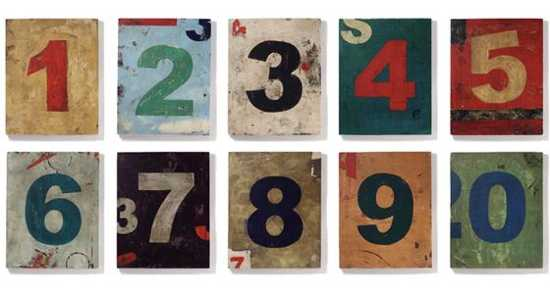A Plank length is extremely small, approximately 1.616199 x 10-35 meters, or in long form 0.00000000000000000000000000000616199 meters. There are about a googol of them in a 1 inch cube. Plank length and Plank volumes are important in quantum physics branches like string theory – evidently sizes this small allow the extra dimensions to be detected, at least in some theories. How do all these small things apply to the third smallest number of this list? There are approximately 8.5 x 10^185 plank volumes in the universe. This number is both massive and its practical purpose relatively non-existent, however it is still simple compared to the rest of the numbers on this list.

7

2^43,112,609 – 1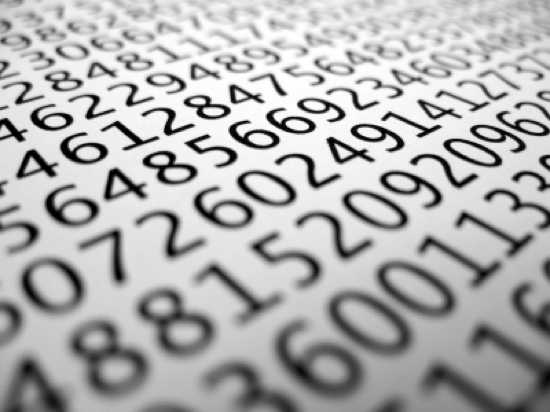The third largest number on this list, the number of all the plank volumes in the universe, consists of 185 digits. This number here consists of almost 13 million digits. The significance of this number is that it is currently the largest known prime number. It was discovered in August of 2008 by the Great Internet Messene Prime Search (GIMPS). From here on the numbers become much more difficult to render.

6

Googolplex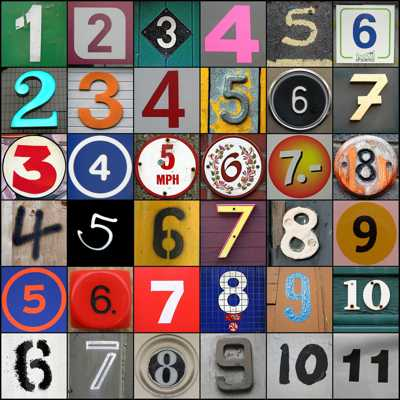A lot of people have heard this word as well, fans of the Back to the Future movies may remember Dr. Emit L. Brown muttering the line “she’s one in a million, one in a billion, one in a googolplex.” But what is a googolplex? Remember how long a googol is? A one with a hundred zeros after it, a googolplex is a 1 with a googol zeros after it. How big is this number? If the entire universe was filled with paper and all that those papers had written on them were zeros at a size 10 font, it would only be about half the zeros required to write out this number in the long form. Even writing the number in scientific notation is not very practical, for a number this large it requires yet another type of notation, something called a power tower. For example our first number 10^80 is the first part of a power tower, as the power tower grows the next number would be placed as a superscript above and to the right of the 80. These are not always possible to write in digital text, so we have to use yet another short hand, the same method used on a graphing calculator, the symbol “^”. So item 10 on this list can be rendered as so 10^80, or ten to the eightieth. Now with this form of notation we can more easily write out the googolplex, which is 10^10^100, or ten to the tenth to the one hundredth. We will also be using these towers for the next few numbers so I hope you’re okay with conceptualizing them.

5

Skewe’s numbers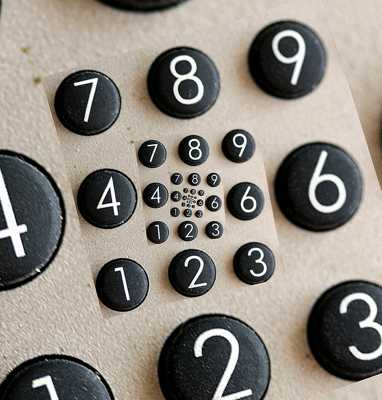Skewe’s Number is the upper bounds to the math problem that: π(x) > Li(x), a simple looking enough equation, however Li is a much more complicated equation in it’s own right. Essentially Skewe’s number proves that a number “x” exists that violates this rule, assuming that Reimanns hypothesis is true then that number “x” is less than 10^10^10^36, (most numbers are) the first of Skewe’s Numbers, much bigger than a googolplex, noted because of the extra tower. There is also an even large Skewe number, without assuming Reimanns hypothesis, x is less then 10^10^10^963.

4

Poincare Recurrence TimeThis is very complicated stuff, but the core concept is relatively simple: “given enough time, anything is possible” Poincare recurrence time is the amount of time that it would take for the entire universe to return to a state that is relatively the same as to what it is today, caused by random quantum fluctuations, or in more simplified terms, “History will repeat itself.” The high estimate of how long this will take is 10^10^10^10^10^1.1 years.

3

Graham’s NumberThis number is massive – in the 1980s it was listed in the Guinness book of world records as the most massive finite number ever used in a serious mathematical proof. It was created Ron Graham, as the upper bounds to a problem in Ramsey Theory involving multicolored hyper cubes. The number is so big even a power tower would be too cumbersome to represent the number. The only way to easily represent the number is to use Knuth’s Up-Arrow Notation and it’s own equation. Let’s go through this piece by piece.

First Knuth’s Up-Arrow Notation is a method of writing very large numbers, it would be much too complicated to explain exactly how the arrows work here, but you can visualize it this way. 3↑3 translates to 33 or 27, 3↑↑3 translates to 3^3^3 or 7,625,597,484,987. Now if you were to add another arrow to the number 3↑↑↑3, then the power tower would be over 7.5 trillion levels. This alone is much much bigger than the Poincare Recurrence time, and you can add an infinite amount of arrows and each arrow makes the number that much more powerful.

The representation of Graham’s number is: G=f64(4), where f(n)=3↑^n3. The best way to look at this is in layers. The first layer is 3↑↑↑↑3, which is already a number too massive to represent in most other forms. The next layer has that many arrows between 3s. Then take that answer and put that many arrows into the next layer between 3s, and this goes on for 64 layers. If you’re interested the last ten digits of Grahams Number are 2464195387, no one, not even Graham himself knows what the first digit is.

2

∞ – Infinity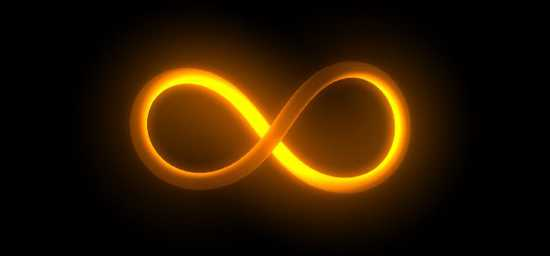Most people know of this number and it is used in hyperbole all the time – kind of like the number one zillion – but it’s more complicated then most people realize, and if you thought the numbers that came before this one were strange, this one is even stranger, and a controversial number too. According to the rules of infinity, there are an infinite number of odd numbers and even numbers in infinity even though there can only be half as many odd numbers as total numbers. Infinity plus one equals infinity, infinity minus one equals infinity, infinity plus infinity equals infinity, infinity divided in half is still infinity, but infinity minus infinity is not exactly understood, infinity divided by infinity would probably be 1.

Scientists estimate 1080 subatomic particles in our known universe, but that is the known universe, or the observable universe. A lot of scientists, however, believe that the universe is infinite, or if they don’t believe this is the case they still accept it as a possibility. If this is the case then by mathematics alone there must be another Earth out there where every atom happens to be in the exact same location in relation to every other atom on Earth as it is in our own. The chances of two carbon copy Earths are extremely small, however in an infinite universe it not only can occur but it has to occur, and not only that, there has to be an infinite amount of carbon copy Earths out there if the universe does in fact go one forever.

Not all people believe in infinity however, Israeli Mathematics Professor Doron Zeilberger, stated that he feels numbers do not go on forever and there is a number so large that when you add 1 to it you will go back to zero, however this number is much higher then anything humans can comprehend, and that number may never be found or proven, this belief is the main pillar in a mathematical philosophy known as Ultrafinitism.

1

∞+1 – Infinity + 1